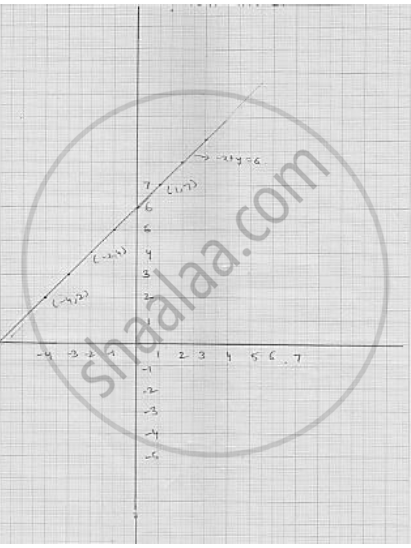# Draw the graph of the following linear equation in two variable : –x + y = 6 - Mathematics

Draw the graph of the following linear equation in two variable : –x + y = 6

#### Solution

We have
- x + y = 6

⇒ x = 6 + x

Putting y  = - 4 , we get  y  = 6 - 4 = 2
Putting  x = -  3  we get y = 6 - 3 = 3
Thus, we get the following table giving the two points on the line represented by the
equation  - x + y = 6
Graph for the equation  - x + y = 6Concept: Graph of a Linear Equation in Two Variables
Is there an error in this question or solution?

#### APPEARS IN

RD Sharma Mathematics for Class 9
Chapter 7 Linear Equations in Two Variables
Exercise 7.3 | Q 1.3

Share